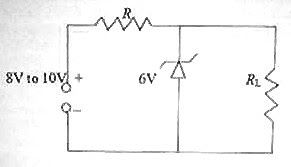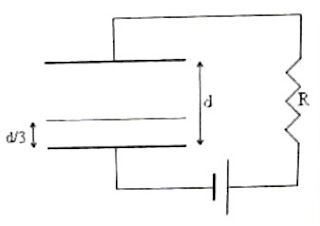## Sunday, June 22, 2008

### Electronics: Multiple Choice Questions on Zener Diodes

In electronics, occasionally you will get questions meant for high lighting the principle of operation and use of Zener diodes. Questions in this section are usually simple. Consider the following question which appeared in KEAM (Medical) 2008 question paper:

In the given circuit, the current through the resistor 2 kΩ is(a) 2 mA

(b) 4 mA

(c) 6 mA

(d) 1 mA

(e) 10 mA

This is a very simple question. The voltage across the reverse biased Zener diode is its breakdown voltage, which is indicated in the figure as 12 V. The load resistor 2 kΩ is connected across the Zener diode and hence the current through it is 12 V/2 kΩ = 6 mA.

Now consider the following question pertaining to the points you have to remember in using a Zener diode as a simple shunt voltage regulator (of the type in the above question):

In the simple shunt voltage regulator circuit shown in the adjoining figure, the unregulated supply voltage varies between 8 V and 10 V. If the maximum reverse current that the Zener diode can safely handle is 100 mA and the Zener diode should draw a minimum current of 5mA (for reliable operation of the voltage regulator), what is the expected maximum current drawn by the load resistor RL?

(a) 95 mA

(b) 105 mA

(c) 200 mA

(d) 10 mA

(e) 110 mA

Since the maximum current allowed through the Zener diode is 100 mA, the maximum current drawn by the Zener diode and the load resistor together is 100 mA itself. If the load is disconnected, the entire current will flow through the Zener diode and this is to be limited to 100 mA.

As the Zener diode is expected to draw at least 5 mA (which happens when the load draws the maximum current), the maximum expected load current is (100 mA – 5 mA) = 95 mA.

[Note that in the shunt voltage regulator, the Zener diode and the load together draws the same current irrespective of the input voltage variation and the load current variation (within the design limits)].

In the above question, what is the value of the series resistance R?

(a) 10 Ω

(b) 20 Ω

(c) 40 Ω

(d) 60 Ω

(e) 95 Ω

You have to consider the maximum unregulated input voltage to determine the value of R. Here it is 10 V. Since the regulated voltage (break down voltage of the Zener diode) is 6 V, the voltage drop across R must be 10 V– 6 V = 4 V.

Since the current through R in all situations (within design limits) is 100 mA, the value of R is given by

R = 4 V/ 100 mA = 4 V/ 0.1 A = 40 Ω.

## Wednesday, June 11, 2008

### IIT-JEE 2008 Multiple Choice Questions on Electrostatics

The following two questions from Electrostatics were included under Straight Objective Type (Multiple choice, single answer type) in the IIT-JEE 2008 question paper:

(1) Consider a system of three charges q/3, q/3 and –2q/3 placed at points A, B and C respectively as shown in the figure. Take O to be the centre of the circle of radius R and angle CAB = 60º(a) The electric field at point O is q/8πε0R2

(b) The potential energy of the system is zero

(c) The magnitude of the force between the charges at C and B is q2/54πε0R2

(d) The potential at point O is q/12πε0R

The electric field at O is due to the negative charge at C only since the equal positive charges situated at A and B will produce equal and opposite fields at O (and they will mutually cancel). The field at O is therefore negative and the option (a) is obviously wrong. Option (b) also is obviously wrong.

The magnitude of the force (F) between the charges at C and B is given by

F = (1/4πε0) [(2q/3)(q/3)/(2Rsin60º)2].

Thus F = q2/54πε0R2 as given in option (c).(2) A parallel plate capacitor with plates of unit area and separation d is filled with a liquid of dielectric constant K = 2. The level of liquid is d/3 initially. Suppose the liquid level decreases at a constant speed V. The time constant as a function of time t is

(a) 6ε0R/(5d + 3Vt)

(b) (15d + 9Vt) ε0R/(2d2 – 3dVt – 9V2t2)

(c) 6ε0R/(5d 3Vt)

(d) (15d – 9Vt) ε0R/(2d2 + 3dVt – 9V2t2)

When a dielectric slab of thickness t1 and dielectric constant K is introduced in between the plates of a parallel plate air capacitor of plate area A and plate separation d, the effective capacitance changes from ε0A/d to ε0A/[dt1 + (t1/K)]

The effective capacitance C of a parallel plate capacitor with parallel dielectric slabs of thickness t1, t2, t3 etc. of dielectric constants K1, K2, K3 etc. respectively is given by the series combined value of capacitors with these dielectrics. Therefore,

1/C = 1/(K1ε0A/t1) + 1/(K2ε0A/t2) + 1/(K3ε0A/t3) + etc.

With a single slab of thickness t1 and dielectric constant K introduced between the plates, we have

1/C = 1/(Kε0A/t1) + 1/[ε0A/(d t1)] from which C = ε0A/[dt1 + (t1/K)]

In the present problem, the thickness of the layer of liquid (which serves as the dielectric slab) at the instant t is (d/3) V t and the thickness of the air space is (2d/3) + V t.

The effective capacitance is therefore given by

C = ε0A/[{(2d/3) + Vt} + {(d/3) Vt}/k)]

Substituting for A = 1 and K = 2, we obtain C = 6ε0/(5d + 3Vt).

The time constant of the circuit = RC = 6ε0R/(5d + 3Vt).

`Reality is merely an illusion, albeit a very persistent one. `
`–Albert Einstein `

## Thursday, June 05, 2008

### All India Pre-Medical/Pre-Dental Entrance Examination (AIPMT) 2008 Questions on Rotational Motion

The following questions appeared in All India Pre-Medical/Pre-Dental Entrance Examination (AIPMT) 2008 question paper:

(1) A thin rod of length L and mass M is bent at its mid point into two halves so that the angle between them is 90º. The moment of inertia of the bent rod about an axis passing through the bending point and perpendicular to the plane defined by the two halves of the rod is

(a) ML2/12

(b) ML2/6

(c) √2 ML2/24

(d) ML2/24

You should note that moment of inertia is a scalar quantity so that the total moment of inertia of the bent rod is the sum of the moments of inertia of the two halves about the common axis. [The axis must be the same when you add the moments of inertia, for obvious reasons].

The moment of inertia of each half is (M/2)(L/2)2/3 so that the moment of inertia of the entire bent rod is 2×[(M/2)(L/2)2]/3 = ML2/12

[You will certainly remember that the moment of inertia of a thin uniform rod of mass m and length about a perpendicular axis through its mid point is m2/12. The moment of inertia about a perpendicular axis through the end, as give by the parallel axis theorem is (m2/12) + m(/2)2 = m2/3]

(2) The ratio of the radii of gyration of a circular disc to that of a circular ring, each of the same mass and radius, about their respective axes is

(a) 1 : √2

(b) √2 : 1

(c) √2 : √3

(d) √3 : √2

Since the moments of inertia of circular disc and circular ring about their axes are respectively MR2/2 and MR2, their radii of gyration about their axes are R/√2 and R respectively.

[Remember that I = Mk2 where I is the moment of inertia and k is the radius of gyration].

Therefore, the ratio of the radii of gyration = (R/√2)/ R = 1/√2.

(3) A roller coaster is designed such that the riders experience “weightlessness” as they go round the top of a hill whose radius of curvature is 20 m. The speed of the car at the top of the hill is between

(a) 15 m/s and 16 m/s

(b) 16 m/s and 17 m/s

(c) 13 m/s and 14 m/s

(d) 14 m/s and 15 m/s

When the rider feels weightless, the weight of the rider is equal in magnitude to the centrifugal force.

Therefore, we have mg = mv2/r where m is the mass of the rider, v is the velocity and r is the radius of the path. From this

v = √(rg) = √(20×10) = √200 = 14.14 m/s.

The correct option therefore is (4).

You can find all posts related to rotational motion on this site by clicking on the label ‘rotational motion’ below this post.

You will find a useful post on the equations to be remembered in rotational motion and circular motion at aphysicsresources